Sec Hotspot 首页  排行榜  收藏本站  技术博客  RSS【安全风险通告】XXL-JOB未授权远程命令执行漏洞安全风险通告   2020.10.27 19:22:04  132禅道开源版高危漏洞风险提示   2020.10.26 10:57:58  105CVE-2020-16898 Windows tcp/ip远程代码执行漏洞复现   2020.10.25 15:00:22  94【全网首发】腾讯商业数据分析师培养计划   2020.10.27 20:17:46  89Windows 内核提权漏洞分析:CVE-2020-1034   2020.10.26 18:00:18  89XXL-JOB 远程命令执行漏洞   2020.10.29 11:32:13  87用Python做数据分析,你还缺几个项目!   2020.10.26 18:51:29  872020看雪SDC议题回顾 | 逃逸IE浏览器沙箱:在野0Day漏洞利用复现   2020.10.26 18:22:03  85今年ByteCTF冠军已被他们“内定”!   2020.10.27 19:16:28  83IDC报告:奇安信天擎EDR获国内市场份额和策略双第一   2020.10.27 19:37:22  79

Galgame汉化中的逆向(三):自定义字库分析0x0 前言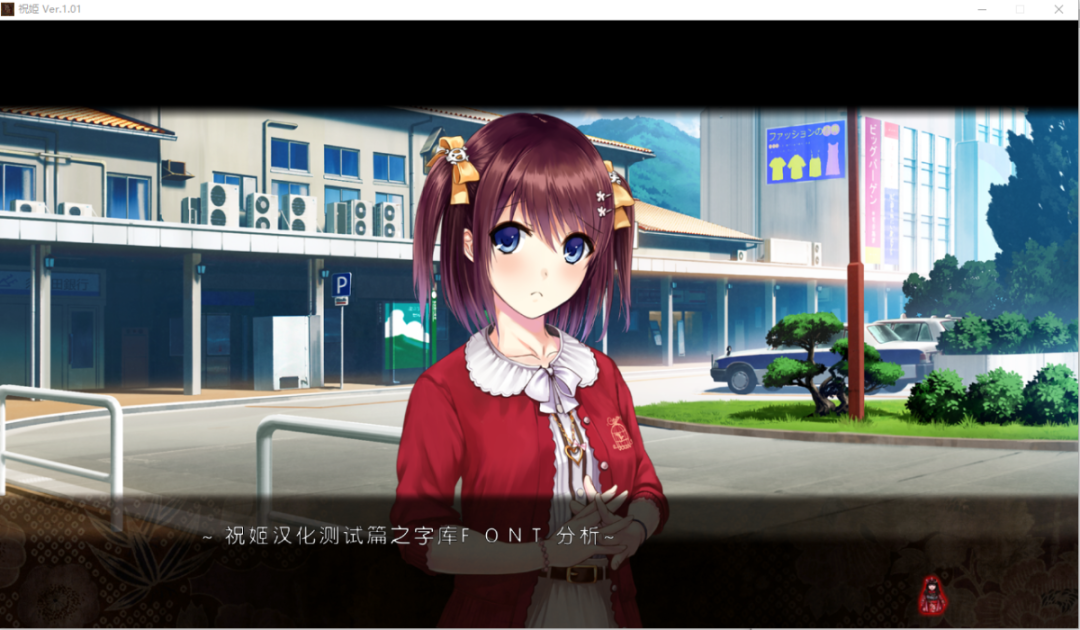0x1 观察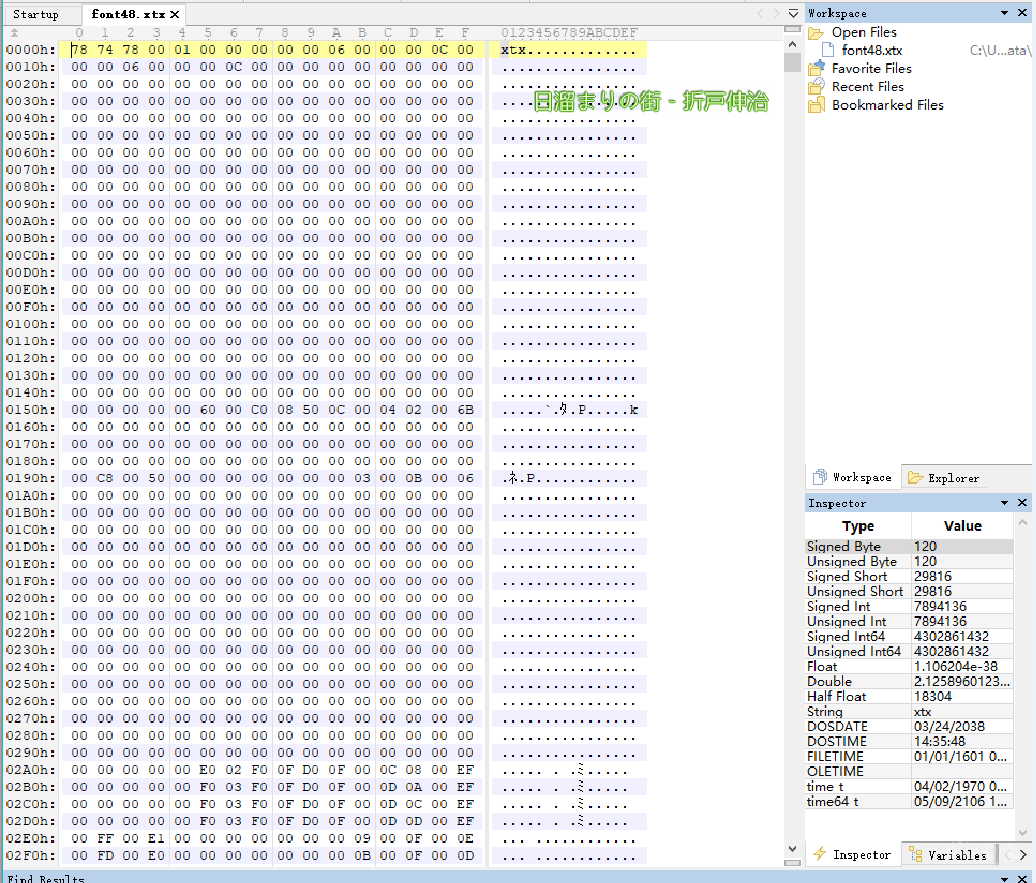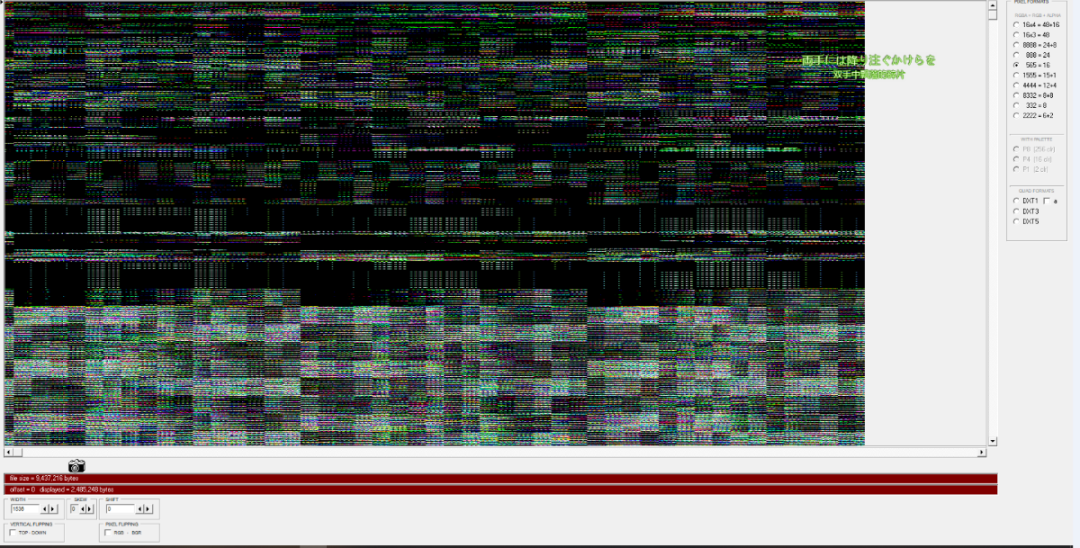0x2 跟踪

0x3 字库分析

``block_size = 48``intensity_map = (``        0b00000000, 0b00010001, 0b00100010, 0b00110011,``        0b01000100, 0b01010101, 0b01100110, 0b01110111,``        0b10001000, 0b10011001, 0b10101010, 0b10111011,``        0b11001100, 0b11011101, 0b11101110, 0b11111111)``def get_x(i, width, level):``    # level means nesting times``    v1 = (level >> 2) + (level >> 1 >> (level >> 2))``    v2 = i << v1``    # 8X8 3f,``    v3 = (v2 & 0x3F) + ((v2 >> 2) & 0x1C0) + ((v2 >> 3) & 0x1FFFFE00)``    return ((((level << 3) - 1) & ((v3 >> 1) ^ ((v3 ^ (v3 >> 1)) & 0xF))) >> v1)+  ((((((v2 >> 6) & 0xFF) + ((v3 >> (v1 + 5)) & 0xFE)) & 3)``            + (((v3 >> (v1 + 7)) % (((width + 31)) >> 5)) << 2)) << 3)``def get_y(i, width, level):``    v1 = (level >> 2) + (level >> 1 >> (level >> 2))``    v2 = i << v1``    v3 = (v2 & 0x3F) + ((v2 >> 2) & 0x1C0) + ((v2 >> 3) & 0x1FFFFE00)``    return ((v3 >> 4) & 1) + ((((v3 & ((level << 6) - 1) & -0x20)``            + ((((v2 & 0x3F)``                + ((v2 >> 2) & 0xC0)) & 0xF) << 1)) >> (v1 + 3)) & -2) + ((((v2 >> 10) & 2) + ((v3 >> (v1 + 6)) & 1)``            + (((v3 >> (v1 + 7)) // ((width + 31) >> 5)) << 2)) << 3)``def xtx_tex12gray(data, height, width, aligned_height, aligned_width):``    gray = np.zeros([width*2, height*2], dtype=np.uint8) # exchange width height``    print("%dX%d xtx, %d bytes-> %dX%d Gray"%(width, height, len(data), height*2, width*2 ))``    for i in range(height*width):``        abs_x = get_x(i, width, 2)``        abs_y = get_y(i, width, 2)``        if abs_y >= height or abs_x >= width:``            continue``        # each 2 byte containes 4 gray pixel``        idx = i * 2``        block_x = (abs_x // block_size) * block_size``        block_y = (abs_y // block_size) * block_size``        x = abs_x % block_size``        y = abs_y % block_size``        target_y = block_y + y``        target_x1 = block_x * 4 + x; # each block(48X48) has 4 cordinate``        target_x2 = block_x * 4 + x + block_size;``        target_x3 = block_x * 4 + x + block_size * 2;``        target_x4 = block_x * 4 + x + block_size * 3;``        gray[target_y][target_x1] = intensity_map[data[idx] >> 4]``        gray[target_y][target_x2] = intensity_map[data[idx] & 0xf]``        gray[target_y][target_x3] = intensity_map[data[idx+1] >> 4]``        gray[target_y][target_x4] = intensity_map[data[idx+1] & 0xf]``    return gray``

``def showtable():``    width = 48``    height = 96``    lines = []``    for i in range(height*2):``        lines.append(width*2*)``    for i in range(width * height):``        x = get_x(i, width, 2)``        y = get_y(i, width, 2)``        lines[y][x] = str(i)``        print(i, y ,x)``    with open('position.csv', 'w', newline='') as fp:``        f_csv = csv.writer(fp)``        f_csv.writerows(lines)``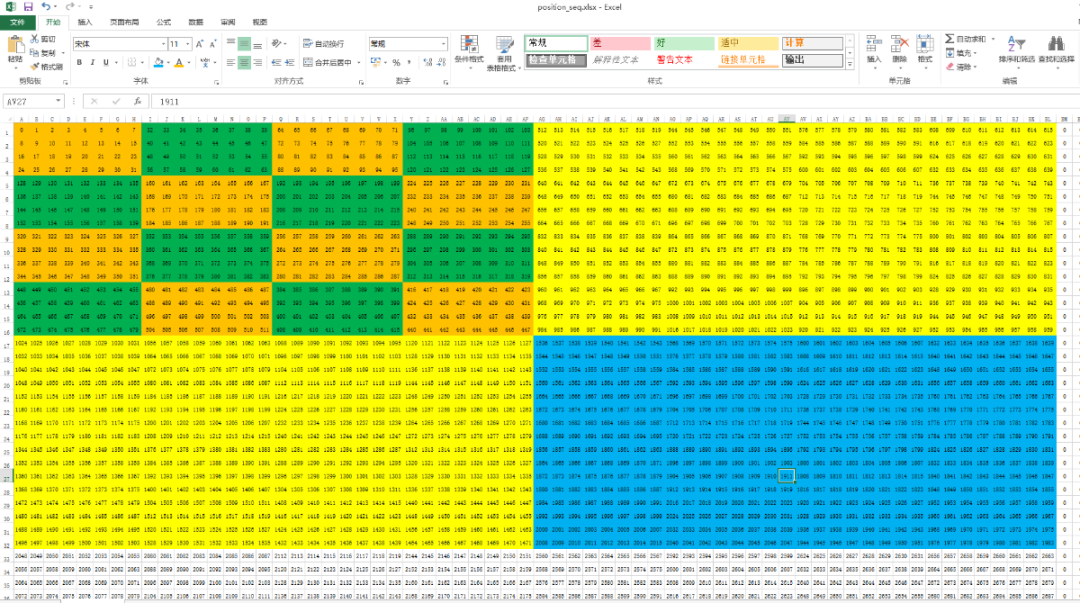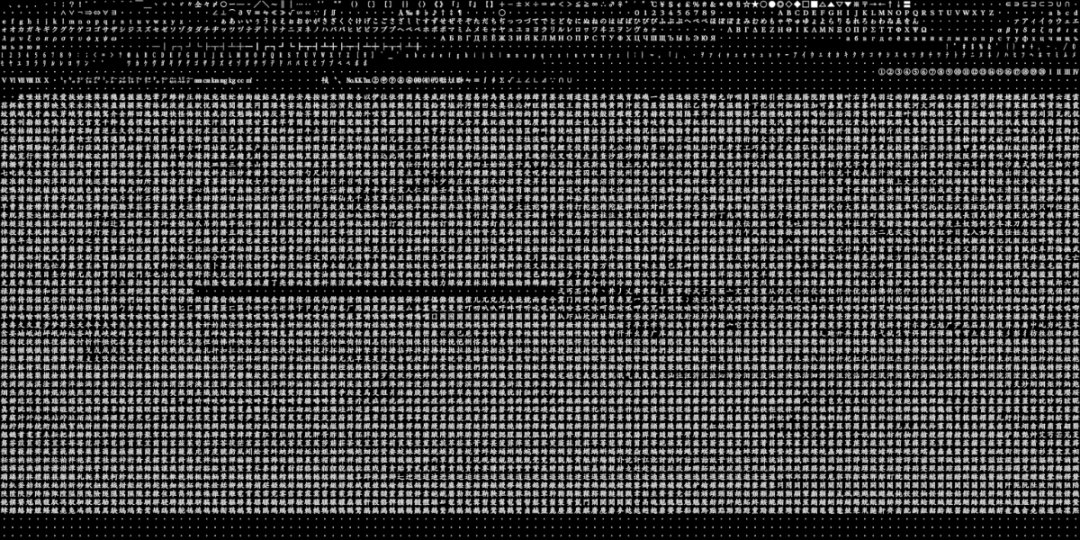0x4 重建字库

（1） 建立简体字库的png图片。

（2）修改fontmap或者将gb2312编码映射到sjis上，生成映射后的码表(.tbl)。

（3）将png转换为该游戏字库格式。

（1）建立图片这个方法太多了，要求是每个字大小48X48，图大小6144X3072。

（2）此游戏的fontmap我没找到，严重怀疑游戏是通过计算来得到的图中位置。 sjis编码的范围是程序里写死的，要改动涉及到的地方太多太麻烦。

（3） 这部解包和封包差不多，只要把src和dst方向对调就行（当然宽高大小之类的参数要微调一下）。

0x5 后记

Galgame汉化本身也是一种有意思的逆向，但是相对来说讨论的不多，我费了好大劲才写完这个帖子，希望能抛砖引玉给大家一些启发吧。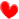- End -https://bbs.pediy.com/user-617776.htm

*本文由看雪论坛 devseed 原创，转载请注明来自看雪社区。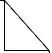ps. 觉得对你有帮助的话，别忘点 分享 点赞 在看 ，支持看雪哦~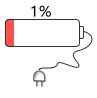“阅读原文 一起来充电吧！